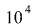• weizhi li

## Homework Statement

a uranium atom traveling at speed 4 *m/s collides elastically with astationary hydrogen molcule. head on. what is the approximate finalspeed of the hydrogen molecule?

## Homework Equations

But, i tried many times and failed.

## The Attempt at a Solution

MY thought is that the speed of uranium atom after collision should be the same but in different direction, which means the momentum of hydrogen after collision is 2*p1(p1 is uranium momentum before collision). and use this to calculate the speed by plugging into the formula k = p^2 / 2m